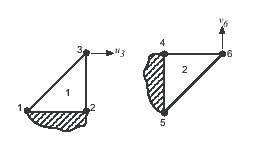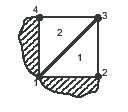# A pathological case of volume locking in triangular elements

Home » Knowledge Base » General » Elements » A pathological case of volume locking in triangular elements

Consider triangle 1, in Figure 1, which is deìned by nodes 1 and 2 on the x axis, and node 3 on the y axis. The area of the triangle is (x2 – x2)y3/2, and it must remain constant if the triangle is incompressible. If nodes 1 and 2 are ixed, then y3 must remain constant and v3 = 0. The remaining degree of freedom is the horizontal displacement u3. Similarly, for the triangle 2, deined by nodes 4, 5, and 6, the only remaining degree of freedom is the vertical displacement v6.

Two triangles may be assembled into a quadrilateral region, see Figure 2. Since incompressibility for triangle 1 requires v4 = 0 and incompressibility for triangle 2 requires u4 = 0, node 4 canet move, and the elements are completely locked up. With nodes 1 through 4 locked up, the nodes for triangles 3 and 4 will also be locked, as will the ones for triangles 5 and 6, see Figure 3. Again, since all previous triangles are locked, adding trianglesFigure 1: The remaining degrees of freedom for two incompressible constant strain triangles.Figure 2: Two incompressible constant strain triangles assembled into a quadrilateral lock.

… 7 and 8 will result in their nodes also being locked. Elements can continue to be added in the same pattern, and all the nodes will be locked. Analogous problems occur in three dimensions with tetrahedral elements.

Locking is eliminated in this situation by using crossed triangles (Figure 4). Although the ìrst quadrilateral generated by crossed triangles has only one degree of freedom, a large assembly of quadrilaterals generated by crossed triangles gives reasonable answers for incompressible problems. Crossed triangles, however, are still overly sti in comparison to correctly formulated quadrilateral elements.